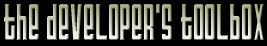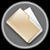This section of the archives stores flipcode's complete Developer Toolbox collection, featuring a variety of mini-articles and source code contributions from our readers.2D OBB Intersection   Submitted byFor 2D graphics or movement of 3D objects on a 2D ground plane it is often useful to discover if the 2D oriented bounding boxes of two objects overlap (have a non-empty intersection). One motivating example is the placement of a new building in a Real-Time Strategy game. The UI needs to continuously check whether the footprint of the new building overlaps the footprint of any existing building. If there is any overlap, the UI should indicate that is an illegal placement. Stefan Gottschalk's thesis (Collision Queries using Oriented Bounding Boxes, Ph.D. Thesis, Department of Computer Science, University of North Carolina at Chapel Hill, 1999) introduces the separating-axis method for performing the equivalent test on 3D oriented bounding boxes. This method depends on the observation that for two boxes to be disjoint (i.e. *not* intersecting), there must be some axis along which their projections are disjoint. The 3D case considers each of 15 axes as a potential separating axis. These axes are the three edge axes of box 1, the three edge axes of box 2, and the nine cross products formed by taking some edge of box 1 and some edge of box 2. In 2D this simplifies dramatically and only four axes need be considered. These are the orthogonal edges of each bounding box. If a few values are precomputed every time a box moves, we end up performing only 16 dot products and some comparisons in the worst case for each overlap test. One nice property of the separating-axis method is that it can be structured in an early out fashion, so that many fewer operations are needed in the case where the boxes do not intersect. In general, the first test is extremely likely to fail (and return "no overlap") when there is no overlap. If it passes, the second test is even more likely to fail if there is no overlap, and so on. Only when the boxes are in extremely close proximity is there even a 50% chance of executing more than 2 tests. The C++ code sample provided efficiently computes this fast 2D oriented bounding box overlap. I augmented the OBB2D class with some methods for rendering and construction to help visualize the result. OBB2D::overlaps1Way performs the real work. It tests to see whether the box passed as an argument overlaps the current box along either of the current box's dimensions. Note that this test must be performed for each box on the other to determine whether there is truly any overlap. To make the tests extremely efficient, OBB2D::origin stores the projection of corner number zero onto a box's axes and the axes are stored explicitly in OBB2D::axis. The magnitude of these stored axes is the inverse of the corresponding edge length so that all overlap tests can be performed on the interval [0, 1] without normalization, and square roots are avoided throughout the entire class.

Download Associated File: 2dobb.h (5,908 bytes)

 ```-------------------------------------------------------------------- 2D OBB IntersectionFor 2D graphics or movement of 3D objects on a 2D ground plane it is often useful to discover if the 2D oriented bounding boxes of two objects overlap (have a non-empty intersection). One motivating example is the placement of a new building in a Real-Time Strategy game. The UI needs to continuously check whether the footprint of the new building overlaps the footprint of any existing building. If there is any overlap, the UI should indicate that is an illegal placement.Stefan Gottschalk's thesis (Collision Queries using Oriented Bounding Boxes, Ph.D. Thesis, Department of Computer Science, University of North Carolina at Chapel Hill, 1999) introduces the separating-axis method for performing the equivalent test on 3D oriented bounding boxes. This method depends on the observation that for two boxes to be disjoint (i.e. *not* intersecting), there must be some axis along which their projections are disjoint. The 3D case considers each of 15 axes as a potential separating axis. These axes are the three edge axes of box 1, the three edge axes of box 2, and the nine cross products formed by taking some edge of box 1 and some edge of box 2. In 2D this simplifies dramatically and only four axes need be considered. These are the orthogonal edges of each bounding box. If a few values are precomputed every time a box moves, we end up performing only 16 dot products and some comparisons in the worst case for each overlap test. One nice property of the separating-axis method is that it can be structured in an early out fashion, so that many fewer operations are needed in the case where the boxes do not intersect. In general, the first test is extremely likely to fail (and return "no overlap") when there is no overlap. If it passes, the second test is even more likely to fail if there is no overlap, and so on. Only when the boxes are in extremely close proximity is there even a 50% chance of executing more than 2 tests.The C++ code sample provided efficiently computes this fast 2D oriented bounding box overlap. I augmented the OBB2D class with some methods for rendering and construction to help visualize the result. OBB2D::overlaps1Way performs the real work. It tests to see whether the box passed as an argument overlaps the current box along either of the current box's dimensions. Note that this test must be performed for each box on the other to determine whether there is truly any overlap. To make the tests extremely efficient, OBB2D::origin stores the projection of corner number zero onto a box's axes and the axes are stored explicitly in OBB2D::axis. The magnitude of these stored axes is the inverse of the corresponding edge length so that all overlap tests can be performed on the interval [0, 1] without normalization, and square roots are avoided throughout the entire class.Morgan McGuire morgan@cs.brown.educlass OBB2D { private: /** Corners of the box, where 0 is the lower left. */ Vector2 corner; /** Two edges of the box extended away from corner. */ Vector2 axis; /** origin[a] = corner.dot(axis[a]); */ double origin; /** Returns true if other overlaps one dimension of this. */ bool overlaps1Way(const OBB2D& other) const { for (int a = 0; a < 2; ++a) { double t = other.corner.dot(axis[a]); // Find the extent of box 2 on axis a double tMin = t; double tMax = t; for (int c = 1; c < 4; ++c) { t = other.corner[c].dot(axis[a]); if (t < tMin) { tMin = t; } else if (t > tMax) { tMax = t; } } // We have to subtract off the origin // See if [tMin, tMax] intersects [0, 1] if ((tMin > 1 + origin[a]) || (tMax < origin[a])) { // There was no intersection along this dimension; // the boxes cannot possibly overlap. return false; } } // There was no dimension along which there is no intersection. // Therefore the boxes overlap. return true; } /** Updates the axes after the corners move. Assumes the corners actually form a rectangle. */ void computeAxes() { axis = corner - corner; axis = corner - corner; // Make the length of each axis 1/edge length so we know any // dot product must be less than 1 to fall within the edge. for (int a = 0; a < 2; ++a) { axis[a] /= axis[a].squaredLength(); origin[a] = corner.dot(axis[a]); } }public: OBB2D(const Vector2& center, const double w, const double h, double angle) { Vector2 X( cos(angle), sin(angle)); Vector2 Y(-sin(angle), cos(angle)); X *= w / 2; Y *= h / 2; corner = center - X - Y; corner = center + X - Y; corner = center + X + Y; corner = center - X + Y; computeAxes(); } /** For testing purposes. */ void moveTo(const Vector2& center) { Vector2 centroid = (corner + corner + corner + corner) / 4; Vector2 translation = center - centroid; for (int c = 0; c < 4; ++c) { corner[c] += translation; } computeAxes(); } /** Returns true if the intersection of the boxes is non-empty. */ bool overlaps(const OBB2D& other) const { return overlaps1Way(other) && other.overlaps1Way(*this); } void render() const { glBegin(GL_LINES); for (int c = 0; c < 5; ++c) { glVertex2fv(corner[c & 3]); } glEnd(); } }; ```

The zip file viewer built into the Developer Toolbox made use of the zlib library, as well as the zlibdll source additions.

Copyright 1999-2008 (C) FLIPCODE.COM and/or the original content author(s). All rights reserved.
Please read our Terms, Conditions, and Privacy information.